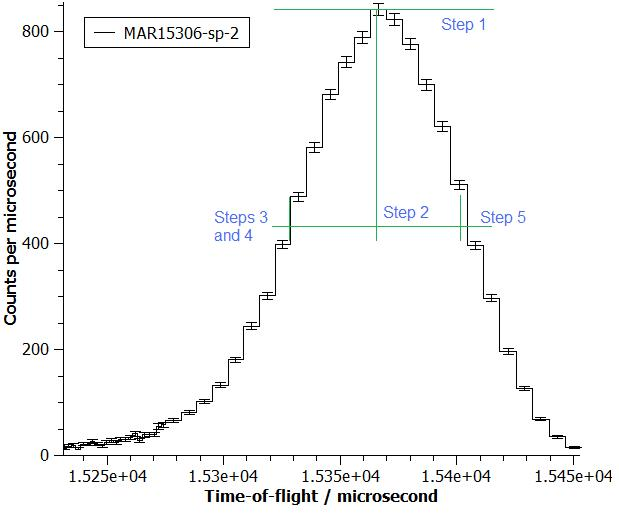$$\renewcommand\AA{\unicode{x212B}}$$

# GetEi v2¶

## Summary¶

Calculates the kinetic energy of neutrons leaving the source based on the time it takes for them to travel between two monitors.

## Properties¶

Name

Direction

Type

Default

Description

InputWorkspace

InOut

MatrixWorkspace

Mandatory

The X units of this workspace must be time of flight with times in microseconds

Monitor1Spec

Input

number

Optional

The spectrum number of the output of the first monitor, e.g. MAPS 41474, MARI 2, MERLIN 69634. If empty, it will be read from the instrument file.

Monitor2Spec

Input

number

Optional

The spectrum number of the output of the second monitor e.g. MAPS 41475, MARI 3, MERLIN 69638. If empty, it will be read from the instrument file.

EnergyEstimate

Input

number

Optional

An approximate value for the typical incident energy, energy of neutrons leaving the source (meV) Can be empty if there is a Sample Log called EnergyRequest. Otherwise it is mandatory.

FixEi

Input

boolean

False

If true, the incident energy will be set to the value of the EnergyEstimate property.

IncidentEnergy

Output

number

The energy of neutron in meV, it is also printed to the Mantid’s log

FirstMonitorPeak

Output

number

The time in $$\rm{\mu s}$$ when the count rate of the first monitor, which defaults to the last monitor the beam hits before the sample, is greatest. It is the mean X value for the bin with the highest number of counts per second and is also writen to Mantid’s log.

FirstMonitorIndex

Output

number

The workspace index of the first monitor in the input workspace.

Tzero

Output

number

PeakSearchRange

Input

number

0.1

Specifies the relative TOF range where the algorithm tries to find the monitor peak. Search occurs within PEAK_TOF_Guess * (1 +/- PeakSearchRange) ranges. Defaults are almost always sufficient but decrease this value for very narrow peaks and increase for wide.

## Description¶

Uses $$E=\frac{1}{2}mv^2$$ to calculate the energy of neutrons leaving the source. The velocity is calculated from the time it takes for the neutron pulse to travel between the two monitors whose spectra were specified. If no spectra are specified, the algorithm will use the defaults for the instrument.

An initial energy guess is required for the algorithm to find the correct peak. The analysis will be done on the highest peak that is within 8% of the estimated TOF given by the estimate. If no initial guess is given, the algorithm will try to get it from the workspace, from a sample log variable called EnergyRequest.

Not all neutrons arrive at the monitors at the same time because their kinetic energies, and therefore velocities, are all different. The time of arrival of the neutron pulse is taken to be the mean of the two half peak height locations. The half height points are found as follows:

1. the peak height is the largest number of counts above the background in any bin in the window

2. the half height is half the above number

3. examine bins to the left of the bin with the highest number of counts looking for a bin with less than half that number above background

4. interpolate between this point bin and the one immediately previous to find the first half height location

5. repeat the steps 3 and 4 looking to the right of the highest point to get the second half height point

6. the mean of the X-values of the two half height points is the TOF arrival time of the neutrons

The above process is illustrated on a peak is shown below in the image below.The distances between the monitors are read from the instrument definition file. It is assumed that the source and the monitors all lie on one line and that the monitors have the same delay time.

## Usage¶

Note

Example: Fixing the Ei

ws = CreateSampleWorkspace(bankPixelWidth=1,binWidth=10)

(ei, firstMonitorPeak, FirstMonitorIndex, tzero) = GetEi(ws,Monitor1Spec=1,Monitor2Spec=2,EnergyEstimate=15.0,FixEi=True)

print("ei: {:.2f}".format(ei))
print("firstMonitorPeak: {:.2f}".format(firstMonitorPeak))
print("FirstMonitorIndex: {}".format(FirstMonitorIndex))
print("tzero: {:.2f}".format(tzero))


Output:

ei: 15.00
firstMonitorPeak: 8854.69
FirstMonitorIndex: 0
tzero: 0.00


ISIS Example

ws = Load("MAR11001.raw")
# Workspace contains monitors
vals = GetEi(ws, EnergyEstimate=12)
# Output from algorithm is a tuple of the following values:
# (IncidentEnergy, FirstMonitorPeak, FirstMonitorIndex, TZero)
print("Calculated Incident Energy = {:.10f} meV".format(vals))
print("First Monitor Peak = {:.8f} microseconds".format(vals))


Output

Calculated Incident Energy = 12.9728953307 meV
First Monitor Peak = 6536.70777852 microseconds


SNS Example

CNCS and HYSPEC do not actually calculate the incident energy, but use the EnergyRequest log value as the calculated incident energy. ARCS and SEQUOIA, however, do perform the calculation for the incident energy. Also, SNS instruments use the negative of the TZero output value in further calculations.

ws = Load("CNCS_7860_event.nxs", LoadMonitors=True)
# Need monitor workspace, as main workspace does not.
# Energy estimate not manditory for SNS instruments
vals = GetEi(ws)
print("Calculated Incident Energy = {:.1f} meV".format(vals))
print("Time Zero = {:.10f} microseconds".format(-vals))


Output:

Calculated Incident Energy = 3.0 meV
Time Zero = -61.7708018029 microseconds


Categories: AlgorithmIndex | Inelastic\Ei